# UMAP降维算法原理详解和应用示例

## (UMAP) 如何工作的？

Projection ——通过投影点在平面、曲面或线上再现空间对象的过程或技术。也可以将其视为对象从高维空间到低维空间的映射。

Approximation——算法假设我们只有一组有限的数据样本（点），而不是构成流形的整个集合。因此，我们需要根据可用数据来近似流形。

Manifold——流形是一个拓扑空间，在每个点附近局部类似于欧几里得空间。一维流形包括线和圆，但不包括类似数字8的形状。二维流形（又名曲面）包括平面、球体、环面等。

Uniform——均匀性假设告诉我们我们的数据样本均匀（均匀）分布在流形上。但是，在现实世界中，这种情况很少发生。因此这个假设引出了在流形上距离是变化的概念。即，空间本身是扭曲的：空间根据数据显得更稀疏或更密集的位置进行拉伸或收缩。

## UMAP执行的步骤

1. 学习高维空间中的流形结构
2. 找到该流形的低维表示。1 — 学习流形结构

1.1.寻找最近的邻居

UMAP 首先使用 Nearest-Neighbor-Descent 算法找到最近的邻居。我们可以通过调整 UMAP 的 n_neighbors 超参数来指定我们想要使用多少个近邻点。

1.2.构建一个图

1.2.1 变化距离1.2.2 local_connectivity

1.2.3 模糊区域

Local_connectivity(默认值为1)：100%确定每个点至少连接到另一个点(连接数量的下限)。

n_neighbors(默认值为15)：一个点直接连接到第 16 个以上的邻居的可能性为 0%，因为它在构建图时落在 UMAP 使用的局部区域之外。

2 到 15 ： 有一定程度的确定性（>0% 但 <100%）一个点连接到它的第 2 个到第 15 个邻居。1.2.4 边的合并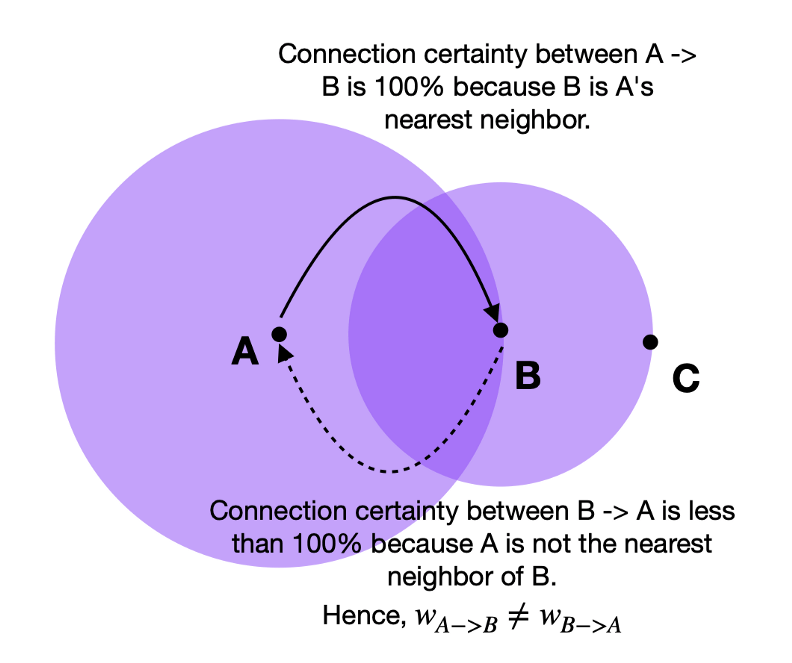UMAP 通过取两条边的并集克服了我们刚刚描述的边权重不一致的问题。 UMAP 文档解释如下：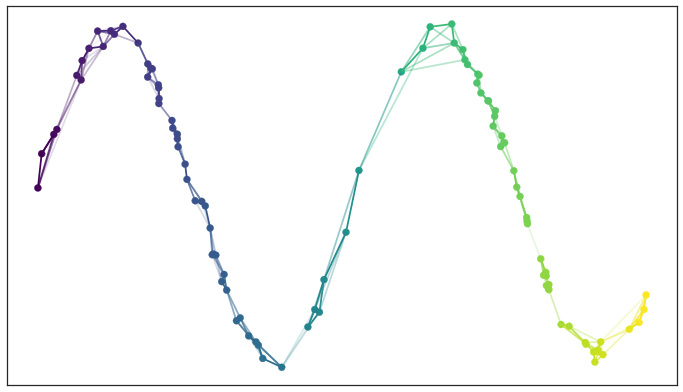2 — 寻找低维表示

2.1.最小距离

2.2.最小化成本函数（Cross-Entropy）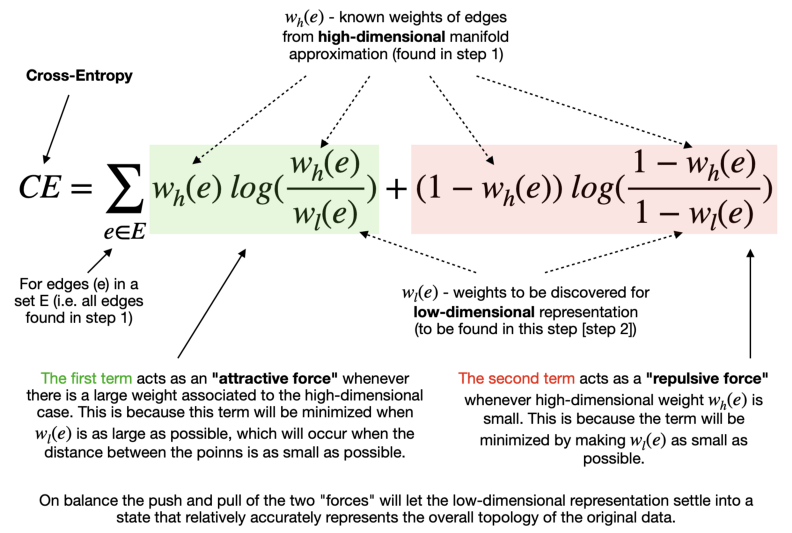## Python中使用UMAP

2、UMAP库执行降维;

3、Plotly和Matplotlib用于数据可视化;

4、Pandas和Numpy用于数据操作。

``````# Data manipulation
import pandas as pd # for data manipulation
import numpy as np # for data manipulation

# Visualization
import plotly.express as px # for data visualization
import matplotlib.pyplot as plt # for showing handwritten digits

# Skleran
from sklearn.datasets import load_digits # for MNIST data
from sklearn.model_selection import train_test_split # for splitting data into train and test samples

# UMAP dimensionality reduction
from umap import UMAP
``````

``````# Load digits data

# Load arrays containing digit data (64 pixels per image) and their true labels

# Some stats
print('Shape of digit images: ', digits.images.shape)
print('Shape of X (main data): ', X.shape)
print('Shape of y (true labels): ', y.shape)

# Display images of the first 10 digits
fig, axs = plt.subplots(2, 5, sharey=False, tight_layout=True, figsize=(12,6), facecolor='white')
n=0
plt.gray()
for i in range(0,2):
for j in range(0,5):
axs[i,j].matshow(digits.images[n])
axs[i,j].set(title=y[n])
n=n+1
plt.show()
``````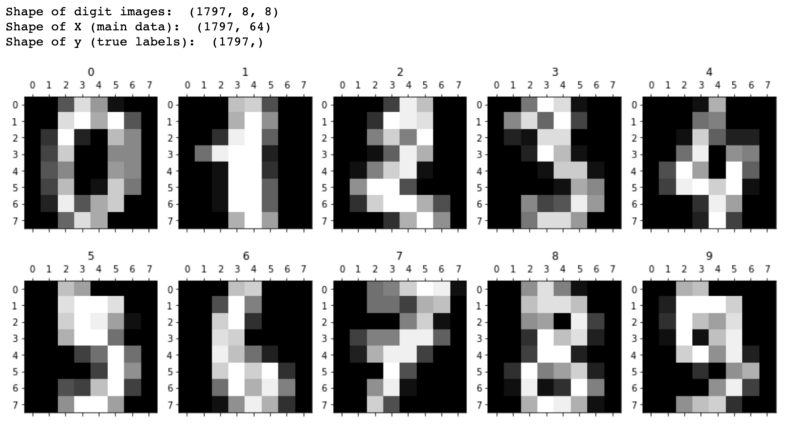``````def chart(X, y):
#--------------------------------------------------------------------------#
# This section is not mandatory as its purpose is to sort the data by label
# so, we can maintain consistent colors for digits across multiple graphs

# Concatenate X and y arrays
arr_concat=np.concatenate((X, y.reshape(y.shape,1)), axis=1)
# Create a Pandas dataframe using the above array
df=pd.DataFrame(arr_concat, columns=['x', 'y', 'z', 'label'])
# Convert label data type from float to integer
df['label'] = df['label'].astype(int)
# Finally, sort the dataframe by label
df.sort_values(by='label', axis=0, ascending=True, inplace=True)
#--------------------------------------------------------------------------#

# Create a 3D graph
fig = px.scatter_3d(df, x='x', y='y', z='z', color=df['label'].astype(str), height=900, width=950)

# Update chart looks
fig.update_layout(title_text='UMAP',
showlegend=True,
legend=dict(orientation="h", yanchor="top", y=0, xanchor="center", x=0.5),
scene_camera=dict(up=dict(x=0, y=0, z=1),
center=dict(x=0, y=0, z=-0.1),
eye=dict(x=1.5, y=-1.4, z=0.5)),
margin=dict(l=0, r=0, b=0, t=0),
scene = dict(xaxis=dict(backgroundcolor='white',
color='black',
gridcolor='#f0f0f0',
title_font=dict(size=10),
tickfont=dict(size=10),
),
yaxis=dict(backgroundcolor='white',
color='black',
gridcolor='#f0f0f0',
title_font=dict(size=10),
tickfont=dict(size=10),
),
zaxis=dict(backgroundcolor='lightgrey',
color='black',
gridcolor='#f0f0f0',
title_font=dict(size=10),
tickfont=dict(size=10),
)))
# Update marker size
fig.update_traces(marker=dict(size=3, line=dict(color='black', width=0.1)))

fig.show()
``````

``````# Configure UMAP hyperparameters
reducer = UMAP(n_neighbors=100, # default 15, The size of local neighborhood (in terms of number of neighboring sample points) used for manifold approximation.
n_components=3, # default 2, The dimension of the space to embed into.
metric='euclidean', # default 'euclidean', The metric to use to compute distances in high dimensional space.
n_epochs=1000, # default None, The number of training epochs to be used in optimizing the low dimensional embedding. Larger values result in more accurate embeddings.
learning_rate=1.0, # default 1.0, The initial learning rate for the embedding optimization.
init='spectral', # default 'spectral', How to initialize the low dimensional embedding. Options are: {'spectral', 'random', A numpy array of initial embedding positions}.
min_dist=0.1, # default 0.1, The effective minimum distance between embedded points.
spread=1.0, # default 1.0, The effective scale of embedded points. In combination with ``min_dist`` this determines how clustered/clumped the embedded points are.
low_memory=False, # default False, For some datasets the nearest neighbor computation can consume a lot of memory. If you find that UMAP is failing due to memory constraints consider setting this option to True.
set_op_mix_ratio=1.0, # default 1.0, The value of this parameter should be between 0.0 and 1.0; a value of 1.0 will use a pure fuzzy union, while 0.0 will use a pure fuzzy intersection.
local_connectivity=1, # default 1, The local connectivity required -- i.e. the number of nearest neighbors that should be assumed to be connected at a local level.
repulsion_strength=1.0, # default 1.0, Weighting applied to negative samples in low dimensional embedding optimization.
negative_sample_rate=5, # default 5, Increasing this value will result in greater repulsive force being applied, greater optimization cost, but slightly more accuracy.
transform_queue_size=4.0, # default 4.0, Larger values will result in slower performance but more accurate nearest neighbor evaluation.
a=None, # default None, More specific parameters controlling the embedding. If None these values are set automatically as determined by ``min_dist`` and ``spread``.
b=None, # default None, More specific parameters controlling the embedding. If None these values are set automatically as determined by ``min_dist`` and ``spread``.
random_state=42, # default: None, If int, random_state is the seed used by the random number generator;
metric_kwds=None, # default None) Arguments to pass on to the metric, such as the ``p`` value for Minkowski distance.
angular_rp_forest=False, # default False, Whether to use an angular random projection forest to initialise the approximate nearest neighbor search.
target_n_neighbors=-1, # default -1, The number of nearest neighbors to use to construct the target simplcial set. If set to -1 use the ``n_neighbors`` value.
#target_metric='categorical', # default 'categorical', The metric used to measure distance for a target array is using supervised dimension reduction. By default this is 'categorical' which will measure distance in terms of whether categories match or are different.
#target_metric_kwds=None, # dict, default None, Keyword argument to pass to the target metric when performing supervised dimension reduction. If None then no arguments are passed on.
#target_weight=0.5, # default 0.5, weighting factor between data topology and target topology.
transform_seed=42, # default 42, Random seed used for the stochastic aspects of the transform operation.
verbose=False, # default False, Controls verbosity of logging.
unique=False, # default False, Controls if the rows of your data should be uniqued before being embedded.
)

# Fit and transform the data
X_trans = reducer.fit_transform(X)

# Check the shape of the new data
print('Shape of X_trans: ', X_trans.shape)
````````````# Split data into training and testing
X_train, X_test, y_train, y_test = train_test_split(X, y, test_size=0.25, shuffle=False)

# Configure UMAP hyperparameters
reducer2 = UMAP(n_neighbors=100, n_components=3, n_epochs=1000,
min_dist=0.5, local_connectivity=2, random_state=42,
)

# Training on MNIST digits data - this time we also pass the true labels to a fit_transform method
X_train_res = reducer2.fit_transform(X_train, y_train)

# Apply on a test set
X_test_res = reducer2.transform(X_test)

# Print the shape of new arrays
print('Shape of X_train_res: ', X_train_res.shape)
print('Shape of X_test_res: ', X_test_res.shape)
````````````chart(X_train_res, y_train)
``````https://chart-studio.plotly.com/create/?fid=SolClover:169

``````chart(X_test_res, y_test)
``````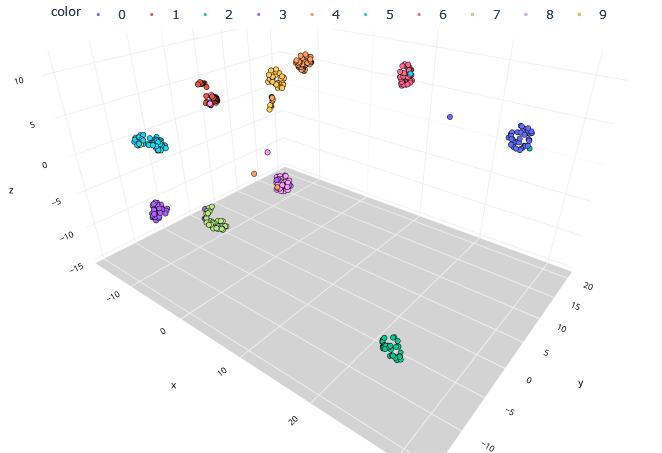https://chart-studio.plotly.com/create/?fid=SolClover:172

## 总结

UMAP的秘诀在于保持低维空间中相对全局距离的同时推断局部和全局结构的能力。这些能力使我们能够找到特定的解决方案，比如找到数字1和2的手写形式之间的相似之处。